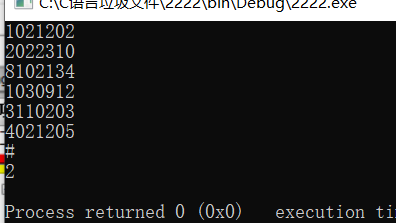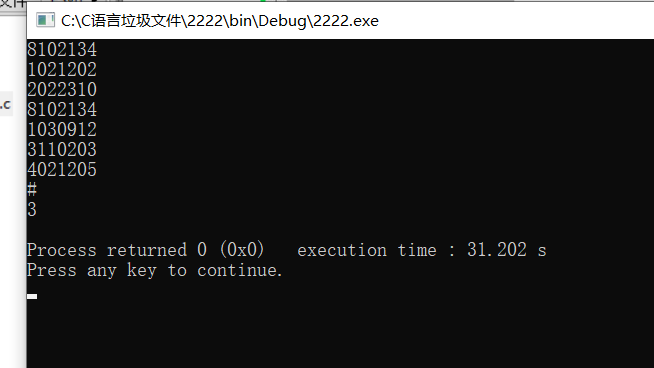，将其中编号为02的计算机专业学生输出。但是我改好后，发现第一个录入的学号无法被使用，如图，改了学号的次序后，结果不一样，求大神解答``````#include <stdio.h>
#include<stdlib.h>
#include<string.h>
struct node
{
char code;
struct node *next;
};
int main()
int i,n,count=0;
char str;
int size=sizeof(struct node);
gets(str);
while(strcmp(str,"#")!=0)
{
p=(struct node*)malloc(size);
strcpy(p->code,str);
gets(str);
}
if(p->code=='0'&&p->code=='2')
count++;
printf("%d\n",count);
return 0;
}
``````

1个回答

gets(str);

while(strcmp(str,"#")!=0)
{
p=(struct node*)malloc(size);
strcpy(p->code,str);
gets(str);
}

for(i=0;i<n;i++)

{
if(p->code=='0'&&p->code=='2')
count++;
p=p->next
}

printf("%d\n",count);
return 0;
}